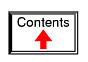# SEQUENTIAL CIRCUITS

*** INCOMPLETE ***

## Binary count sequence

If we examine a four-bit binary count sequence from 0000 to 1111, a definite pattern will be evident in the "oscillations" of the bits between 0 and 1:Note how the least significant bit (LSB) toggles between 0 and 1 for every step in the count sequence, while each succeeding bit toggles at one-half the frequency of the one before it. The most significant bit (MSB) only toggles once during the entire sixteen-step count sequence: at the transition between 7 (0111) and 8 (1000).

If we wanted to design a digital circuit to "count" in four-bit binary, all we would have to do is design a series of frequency divider circuits, each circuit dividing the frequency of a square-wave pulse by a factor of 2: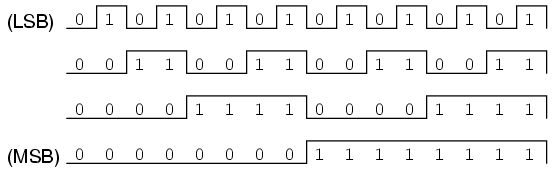J-K flip-flops are ideally suited for this task, because they have the ability to "toggle" their output state at the command of a clock pulse when both J and K inputs are made "high" (1):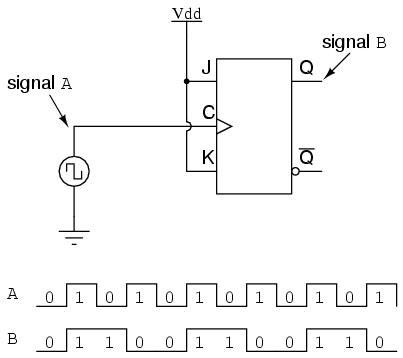If we consider the two signals (A and B) in this circuit to represent two bits of a binary number, signal A being the LSB and signal B being the MSB, we see that the count sequence is backward: from 11 to 10 to 01 to 00 and back again to 11. Although it might not be counting in the direction we might have assumed, at least it counts!

The following sections explore different types of counter circuits, all made with J-K flip-flops, and all based on the exploitation of that flip-flop's toggle mode of operation.

• REVIEW:
• Binary count sequences follow a pattern of octave frequency division: the frequency of oscillation for each bit, from LSB to MSB, follows a divide-by-two pattern. In other words, the LSB will oscillate at the highest frequency, followed by the next bit at one-half the LSB's frequency, and the next bit at one-half the frequency of the bit before it, etc.
• Circuits may be built that "count" in a binary sequence, using J-K flip-flops set up in the "toggle" mode.

## Asynchronous counters

In the previous section, we saw a circuit using one J-K flip-flop that counted backward in a two-bit binary sequence, from 11 to 10 to 01 to 00. Since it would be desirable to have a circuit that could count forward and not just backward, it would be worthwhile to examine a forward count sequence again and look for more patterns that might indicate how to build such a circuit.

Since we know that binary count sequences follow a pattern of octave (factor of 2) frequency division, and that J-K flip-flop multivibrators set up for the "toggle" mode are capable of performing this type of frequency division, we can envision a circuit made up of several J-K flip-flops, cascaded to produce four bits of output. The main problem facing us is to determine how to connect these flip-flops together so that they toggle at the right times to produce the proper binary sequence. Examine the following binary count sequence, paying attention to patterns preceding the "toggling" of a bit between 0 and 1:Note that each bit in this four-bit sequence toggles when the bit before it (the bit having a lesser significance, or place-weight), toggles in a particular direction: from 1 to 0. Small arrows indicate those points in the sequence where a bit toggles, the head of the arrow pointing to the previous bit transitioning from a "high" (1) state to a "low" (0) state:Starting with four J-K flip-flops connected in such a way to always be in the "toggle" mode, we need to determine how to connect the clock inputs in such a way so that each succeeding bit toggles when the bit before it transitions from 1 to 0. The Q outputs of each flip-flop will serve as the respective binary bits of the final, four-bit count:If we used flip-flops with negative-edge triggering (bubble symbols on the clock inputs), we could simply connect the clock input of each flip-flop to the Q output of the flip-flop before it, so that when the bit before it changes from a 1 to a 0, the "falling edge" of that signal would "clock" the next flip-flop to toggle the next bit:This circuit would yield the following output waveforms, when "clocked" by a repetitive source of pulses from an oscillator:The first flip-flop (the one with the Q0 output), has a positive-edge triggered clock input, so it toggles with each rising edge of the clock signal. Notice how the clock signal in this example has a duty cycle less than 50%. I've shown the signal in this manner for the purpose of demonstrating how the clock signal need not be symmetrical to obtain reliable, "clean" output bits in our four-bit binary sequence. In the very first flip-flop circuit shown in this chapter, I used the clock signal itself as one of the output bits. This is a bad practice in counter design, though, because it necessitates the use of a square wave signal with a 50% duty cycle ("high" time = "low" time) in order to obtain a count sequence where each and every step pauses for the same amount of time. Using one J-K flip-flop for each output bit, however, relieves us of the necessity of having a symmetrical clock signal, allowing the use of practically any variety of high/low waveform to increment the count sequence.

As indicated by all the other arrows in the pulse diagram, each succeeding output bit is toggled by the action of the preceding bit transitioning from "high" (1) to "low" (0). This is the pattern necessary to generate an "up" count sequence.

A less obvious solution for generating an "up" sequence using positive-edge triggered flip-flops is to "clock" each flip-flop using the Q' output of the preceding flip-flop rather than the Q output. Since the Q' output will always be the exact opposite state of the Q output on a J-K flip-flop (no invalid states with this type of flip-flop), a high-to-low transition on the Q output will be accompanied by a low-to-high transition on the Q' output. In other words, each time the Q output of a flip-flop transitions from 1 to 0, the Q' output of the same flip-flop will transition from 0 to 1, providing the positive-going clock pulse we would need to toggle a positive-edge triggered flip-flop at the right moment:One way we could expand the capabilities of either of these two counter circuits is to regard the Q' outputs as another set of four binary bits. If we examine the pulse diagram for such a circuit, we see that the Q' outputs generate a down-counting sequence, while the Q outputs generate an up-counting sequence: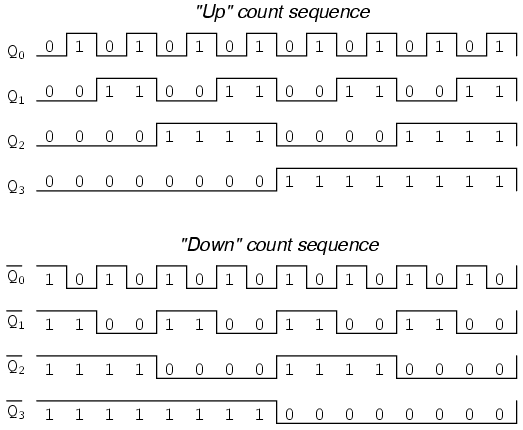Unfortunately, all of the counter circuits shown thusfar share a common problem: the ripple effect. This effect is seen in certain types of binary adder and data conversion circuits, and is due to accumulative propagation delays between cascaded gates. When the Q output of a flip-flop transitions from 1 to 0, it commands the next flip-flop to toggle. If the next flip-flop toggle is a transition from 1 to 0, it will command the flip-flop after it to toggle as well, and so on. However, since there is always some small amount of propagation delay between the command to toggle (the clock pulse) and the actual toggle response (Q and Q' outputs changing states), any subsequent flip-flops to be toggled will toggle some time after the first flip-flop has toggled. Thus, when multiple bits toggle in a binary count sequence, they will not all toggle at exactly the same time: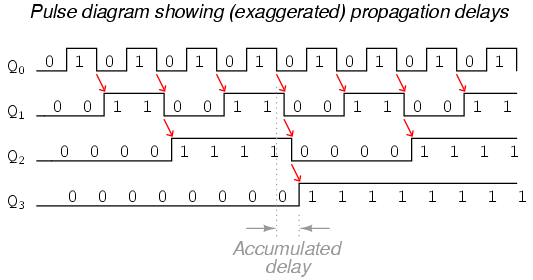As you can see, the more bits that toggle with a given clock pulse, the more severe the accumulated delay time from LSB to MSB. When a clock pulse occurs at such a transition point (say, on the transition from 0111 to 1000), the output bits will "ripple" in sequence from LSB to MSB, as each succeeding bit toggles and commands the next bit to toggle as well, with a small amount of propagation delay between each bit toggle. If we take a close-up look at this effect during the transition from 0111 to 1000, we can see that there will be false output counts generated in the brief time period that the "ripple" effect takes place: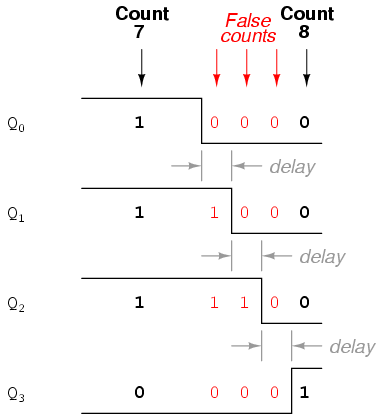Instead of cleanly transitioning from a "0111" output to a "1000" output, the counter circuit will very quickly ripple from 0111 to 0110 to 0100 to 0000 to 1000, or from 7 to 6 to 4 to 0 and then to 8. This behavior earns the counter circuit the name of ripple counter, or asynchronous counter.

In many applications, this effect is tolerable, since the ripple happens very, very quickly (the width of the delays has been exaggerated here as an aid to understanding the effects). If all we wanted to do was drive a set of light-emitting diodes (LEDs) with the counter's outputs, for example, this brief ripple would be of no consequence at all. However, if we wished to use this counter to drive the "select" inputs of a multiplexer, index a memory pointer in a microprocessor (computer) circuit, or perform some other task where false outputs could cause spurious errors, it would not be acceptable. There is a way to use this type of counter circuit in applications sensitive to false, ripple-generated outputs, and it involves a principle known as strobing.

Most decoder and multiplexer circuits are equipped with at least one input called the "enable." The output(s) of such a circuit will be active only when the enable input is made active. We can use this enable input to strobe the circuit receiving the ripple counter's output so that it is disabled (and thus not responding to the counter output) during the brief period of time in which the counter outputs might be rippling, and enabled only when sufficient time has passed since the last clock pulse that all rippling will have ceased. In most cases, the strobing signal can be the same clock pulse that drives the counter circuit: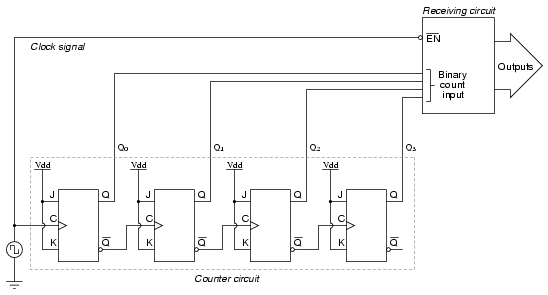With an active-low Enable input, the receiving circuit will respond to the binary count of the four-bit counter circuit only when the clock signal is "low." As soon as the clock pulse goes "high," the receiving circuit stops responding to the counter circuit's output. Since the counter circuit is positive-edge triggered (as determined by the first flip-flop clock input), all the counting action takes place on the low-to-high transition of the clock signal, meaning that the receiving circuit will become disabled just before any toggling occurs on the counter circuit's four output bits. The receiving circuit will not become enabled until the clock signal returns to a low state, which should be a long enough time after all rippling has ceased to be "safe" to allow the new count to have effect on the receiving circuit. The crucial parameter here is the clock signal's "high" time: it must be at least as long as the maximum expected ripple period of the counter circuit. If not, the clock signal will prematurely enable the receiving circuit, while some rippling is still taking place.

Another disadvantage of the asynchronous, or ripple, counter circuit is limited speed. While all gate circuits are limited in terms of maximum signal frequency, the design of asynchronous counter circuits compounds this problem by making propagation delays additive. Thus, even if strobing is used in the receiving circuit, an asynchronous counter circuit cannot be clocked at any frequency higher than that which allows the greatest possible accumulated propagation delay to elapse well before the next pulse.

The solution to this problem is a counter circuit that avoids ripple altogether. Such a counter circuit would eliminate the need to design a "strobing" feature into whatever digital circuits use the counter output as an input, and would also enjoy a much greater operating speed than its asynchronous equivalent. This design of counter circuit is the subject of the next section.

• REVIEW:
• An "up" counter may be made by connecting the clock inputs of positive-edge triggered J-K flip-flops to the Q' outputs of the preceding flip-flops. Another way is to use negative-edge triggered flip-flops, connecting the clock inputs to the Q outputs of the preceding flip-flops. In either case, the J and K inputs of all flip-flops are connected to Vcc or Vdd so as to always be "high."
• Counter circuits made from cascaded J-K flip-flops where each clock input receives its pulses from the output of the previous flip-flop invariably exhibit a ripple effect, where false output counts are generated between some steps of the count sequence. These types of counter circuits are called asynchronous counters, or ripple counters.
• Strobing is a technique applied to circuits receiving the output of an asynchronous (ripple) counter, so that the false counts generated during the ripple time will have no ill effect. Essentially, the enable input of such a circuit is connected to the counter's clock pulse in such a way that it is enabled only when the counter outputs are not changing, and will be disabled during those periods of changing counter outputs where ripple occurs.

## Synchronous counters

A synchronous counter, in contrast to an asynchronous counter, is one whose output bits change state simultaneously, with no ripple. The only way we can build such a counter circuit from J-K flip-flops is to connect all the clock inputs together, so that each and every flip-flop receives the exact same clock pulse at the exact same time: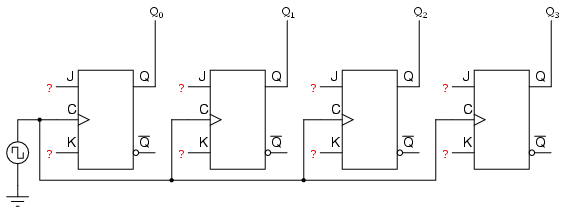Now, the question is, what do we do with the J and K inputs? We know that we still have to maintain the same divide-by-two frequency pattern in order to count in a binary sequence, and that this pattern is best achieved utilizing the "toggle" mode of the flip-flop, so the fact that the J and K inputs must both be (at times) "high" is clear. However, if we simply connect all the J and K inputs to the positive rail of the power supply as we did in the asynchronous circuit, this would clearly not work because all the flip-flops would toggle at the same time: with each and every clock pulse!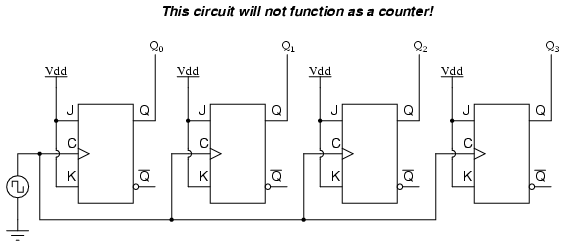Let's examine the four-bit binary counting sequence again, and see if there are any other patterns that predict the toggling of a bit. Asynchronous counter circuit design is based on the fact that each bit toggle happens at the same time that the preceding bit toggles from a "high" to a "low" (from 1 to 0). Since we cannot clock the toggling of a bit based on the toggling of a previous bit in a synchronous counter circuit (to do so would create a ripple effect) we must find some other pattern in the counting sequence that can be used to trigger a bit toggle:

Examining the four-bit binary count sequence, another predictive pattern can be seen. Notice that just before a bit toggles, all preceding bits are "high:"This pattern is also something we can exploit in designing a counter circuit. If we enable each J-K flip-flop to toggle based on whether or not all preceding flip-flop outputs (Q) are "high," we can obtain the same counting sequence as the asynchronous circuit without the ripple effect, since each flip-flop in this circuit will be clocked at exactly the same time: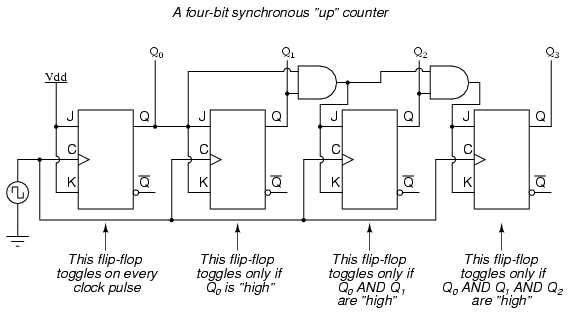The result is a four-bit synchronous "up" counter. Each of the higher-order flip-flops are made ready to toggle (both J and K inputs "high") if the Q outputs of all previous flip-flops are "high." Otherwise, the J and K inputs for that flip-flop will both be "low," placing it into the "latch" mode where it will maintain its present output state at the next clock pulse. Since the first (LSB) flip-flop needs to toggle at every clock pulse, its J and K inputs are connected to Vcc or Vdd, where they will be "high" all the time. The next flip-flop need only "recognize" that the first flip-flop's Q output is high to be made ready to toggle, so no AND gate is needed. However, the remaining flip-flops should be made ready to toggle only when all lower-order output bits are "high," thus the need for AND gates.

To make a synchronous "down" counter, we need to build the circuit to recognize the appropriate bit patterns predicting each toggle state while counting down. Not surprisingly, when we examine the four-bit binary count sequence, we see that all preceding bits are "low" prior to a toggle (following the sequence from bottom to top):Since each J-K flip-flop comes equipped with a Q' output as well as a Q output, we can use the Q' outputs to enable the toggle mode on each succeeding flip-flop, being that each Q' will be "high" every time that the respective Q is "low:"Taking this idea one step further, we can build a counter circuit with selectable between "up" and "down" count modes by having dual lines of AND gates detecting the appropriate bit conditions for an "up" and a "down" counting sequence, respectively, then use OR gates to combine the AND gate outputs to the J and K inputs of each succeeding flip-flop:This circuit isn't as complex as it might first appear. The Up/Down control input line simply enables either the upper string or lower string of AND gates to pass the Q/Q' outputs to the succeeding stages of flip-flops. If the Up/Down control line is "high," the top AND gates become enabled, and the circuit functions exactly the same as the first ("up") synchronous counter circuit shown in this section. If the Up/Down control line is made "low," the bottom AND gates become enabled, and the circuit functions identically to the second ("down" counter) circuit shown in this section.

To illustrate, here is a diagram showing the circuit in the "up" counting mode (all disabled circuitry shown in grey rather than black):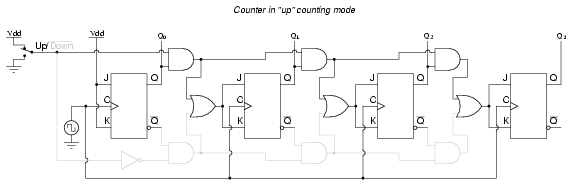Here, shown in the "down" counting mode, with the same grey coloring representing disabled circuitry: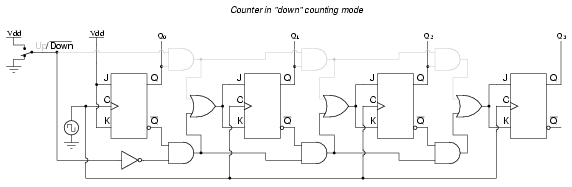Up/down counter circuits are very useful devices. A common application is in machine motion control, where devices called rotary shaft encoders convert mechanical rotation into a series of electrical pulses, these pulses "clocking" a counter circuit to track total motion: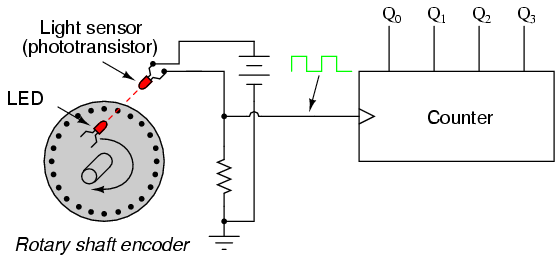As the machine moves, it turns the encoder shaft, making and breaking the light beam between LED and phototransistor, thereby generating clock pulses to increment the counter circuit. Thus, the counter integrates, or accumulates, total motion of the shaft, serving as an electronic indication of how far the machine has moved. If all we care about is tracking total motion, and do not care to account for changes in the direction of motion, this arrangement will suffice. However, if we wish the counter to increment with one direction of motion and decrement with the reverse direction of motion, we must use an up/down counter, and an encoder/decoding circuit having the ability to discriminate between different directions.

If we re-design the encoder to have two sets of LED/phototransistor pairs, those pairs aligned such that their square-wave output signals are 90o out of phase with each other, we have what is known as a quadrature output encoder (the word "quadrature" simply refers to a 90o angular separation). A phase detection circuit may be made from a D-type flip-flop, to distinguish a clockwise pulse sequence from a counter-clockwise pulse sequence: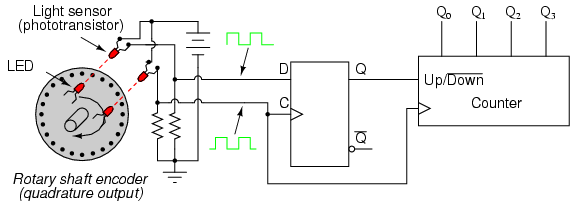When the encoder rotates clockwise, the "D" input signal square-wave will lead the "C" input square-wave, meaning that the "D" input will already be "high" when the "C" transitions from "low" to "high," thus setting the D-type flip-flop (making the Q output "high") with every clock pulse. A "high" Q output places the counter into the "Up" count mode, and any clock pulses received by the clock from the encoder (from either LED) will increment it. Conversely, when the encoder reverses rotation, the "D" input will lag behind the "C" input waveform, meaning that it will be "low" when the "C" waveform transitions from "low" to "high," forcing the D-type flip-flop into the reset state (making the Q output "low") with every clock pulse. This "low" signal commands the counter circuit to decrement with every clock pulse from the encoder.

This circuit, or something very much like it, is at the heart of every position-measuring circuit based on a pulse encoder sensor. Such applications are very common in robotics, CNC machine tool control, and other applications involving the measurement of reversible, mechanical motion.

INCOMPLETE

## Finite State Machines

Up to now, every circuit that was presented was a combinatorial circuit. That means that its output is dependent only by its current inputs. Previous inputs for that type of circuits have no effect on the output.

However, there are many applications where there is a need for our circuits to have "memory"; to remember previous inputs and calculate their outputs according to them. A circuit whose output depends not only on the present input but also on the history of the input is called a sequential circuit.

In this section we will learn how to design and build such sequential circuits. In order to see how this procedure works, we will use an example, on which we will study our topic.

So let's suppose we have a digital quiz game that works on a clock and reads an input from a manual button. However, we want the switch to transmit only one HIGH pulse to the circuit. If we hook the button directly on the game circuit it will transmit HIGH for as few clock cycles as our finger can achieve. On a common clock frequency our finger can never be fast enough.

The desing procedure has specific steps that must be followed in order to get the work done:

Step 1

The first step of the design procedure is to define with simple but clear words what we want our circuit to do:

"Our mission is to design a secondary circuit that will transmit a HIGH pulse with duration of only one cycle when the manual button is pressed, and won't transmit another pulse until the button is depressed and pressed again."

Step 2

The next step is to design a State Diagram. This is a diagram that is made from circles and arrows and describes visually the operation of our circuit. In mathematic terms, this diagram that describes the operation of our sequential circuit is a Finite State Machine.

Make a note that this is a Moore Finite State Machine. Its output is a function of only its current state, not its input. That is in contrast with the Mealy Finite State Machine, where input affects the output. In this tutorial, only the Moore Finite State Machine will be examined.

The State Diagram of our circuit is the following: (Figure below)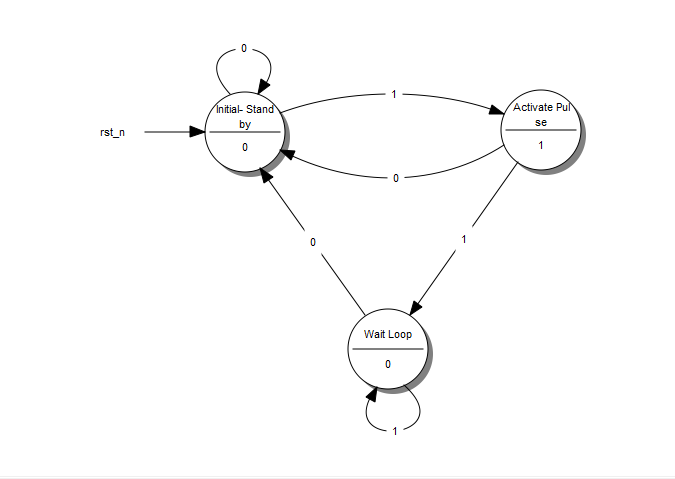A State Diagram

Every circle represents a "state", a well-defined condition that our machine can be found at.

In the upper half of the circle we describe that condition. The description helps us remember what our circuit is supposed to do at that condition.

• The first circle is the "stand-by" condition. This is where our circuit starts from and where it waits for another button press.
• The second circle is the condition where the button has just been just pressed and our circuit needs to transmit a HIGH pulse.
• The third circle is the condition where our circuit waits for the button to be released before it returns to the "stand-by" condition.

In the lower part of the circle is the output of our circuit. If we want our circuit to transmit a HIGH on a specific state, we put a 1 on that state. Otherwise we put a 0.

Every arrow represents a "transition" from one state to another. A transition happens once every clock cycle. Depending on the current Input, we may go to a different state each time. Notice the number in the middle of every arrow. This is the current Input.

For example, when we are in the "Initial-Stand by" state and we "read" a 1, the diagram tells us that we have to go to the "Activate Pulse" state. If we read a 0 we must stay on the "Initial-Stand by" state.

So, what does our "Machine" do exactly? It starts from the "Initial - Stand by" state and waits until a 1 is read at the Input. Then it goes to the "Activate Pulse" state and transmits a HIGH pulse on its output. If the button keeps being pressed, the circuit goes to the third state, the "Wait Loop". There it waits until the button is released (Input goes 0) while transmitting a LOW on the output. Then it's all over again!

This is possibly the most difficult part of the design procedure, because it cannot be described by simple steps. It takes exprerience and a bit of sharp thinking in order to set up a State Diagram, but the rest is just a set of predetermined steps.

Step 3

Next, we replace the words that describe the different states of the diagram with binary numbers. We start the enumeration from 0 which is assigned on the initial state. We then continue the enumeration with any state we like, until all states have their number.

The result looks something like this: (Figure below)A State Diagram with Coded States

Step 4

Afterwards, we fill the State Table. This table has a very specific form. I will give the table of our example and use it to explain how to fill it in. (Figure below)A State Table

The first columns are as many as the bits of the highest number we assigned the State Diagram. If we had 5 states, we would have used up to the number 100, which means we would use 3 columns. For our example, we used up to the number 10, so only 2 columns will be needed. These columns describe the Current State of our circuit.

To the right of the Current State columns we write the Input Columns. These will be as many as our Input variables. Our example has only one Input.

Next, we write the Next State Columns. These are as many as the Current State columns.

Finally, we write the Outputs Columns. These are as many as our outputs. Our example has only one output. Since we have built a More Finite State Machine, the output is dependent on only the current input states. This is the reason the outputs column has two 1: to result in an output Boolean function that is independant of input I. Keep on reading for further details.

The Current State and Input columns are the Inputs of our table. We fill them in with all the binary numbers from 0 to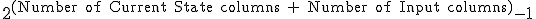It is simpler than it sounds fortunately. Usually there will be more rows than the actual States we have created in the State Diagram, but that's ok.

Each row of the Next State columns is filled as follows: We fill it in with the state that we reach when, in the State Diagram, from the Current State of the same row we follow the Input of the same row. If have to fill in a row whose Current State number doesn't correspond to any actual State in the State Diagram we fill it with Don't Care terms (X). After all, we don't care where we can go from a State that doesn't exist. We wouldn't be there in the first place! Again it is simpler than it sounds.

The outputs column is filled by the output of the corresponding Current State in the State Diagram.

The State Table is complete! It describes the behaviour of our circuit as fully as the State Diagram does.

Step 5a

The next step is to take that theoretical "Machine" and implement it in a circuit. Most often than not, this implementation involves Flip Flops. This guide is dedicated to this kind of implementation and will describe the procedure for both D - Flip Flops as well as JK - Flip Flops. T - Flip Flops will not be included as they are too similar to the two previous cases.

The selection of the Flip Flop to use is arbitrary and usually is determined by cost factors. The best choice is to perform both analysis and decide which type of Flip Flop results in minimum number of logic gates and lesser cost.

First we will examine how we implement our "Machine" with D-Flip Flops.

We will need as many D - Flip Flops as the State columns, 2 in our example. For every Flip Flop we will add one more column in our State table (Figure below) with the name of the Flip Flop's input, "D" for this case. The column that corresponds to each Flip Flop describes what input we must give the Flip Flop in order to go from the Current State to the Next State. For the D - Flip Flop this is easy: The necessary input is equal to the Next State. In the rows that contain X's we fill X's in this column as well.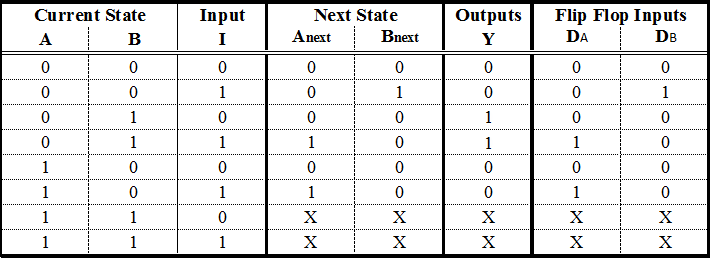A State Table with D - Flip Flop Excitations

Step 5b

We can do the same steps with JK - Flip Flops. There are some differences however. A JK - Flip Flop has two inputs, therefore we need to add two columns for each Flip Flop. The content of each cell is dictated by the JK's excitation table: (Figure below)

JK - Flip Flop Excitation TableThis table says that if we want to go from State Q to State Qnext, we need to use the specific input for each terminal. For example, to go from 0 to 1, we need to feed J with 1 and we don't care which input we feed to terminal K.A State Table with JK - Flip Flop Excitations

Step 6

We are in the final stage of our procedure. What remains, is to determine the Boolean functions that produce the inputs of our Flip Flops and the Output. We will extract one Boolean funtion for each Flip Flop input we have. This can be done with a Karnaugh Map. The input variables of this map are the Current State variables as well as the Inputs.

That said, the input functions for our D - Flip Flops are the following: (Figure below)Karnaugh Maps for the D - Flip Flop Inputs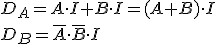If we chose to use JK - Flip Flops our functions would be the following: (Figure below)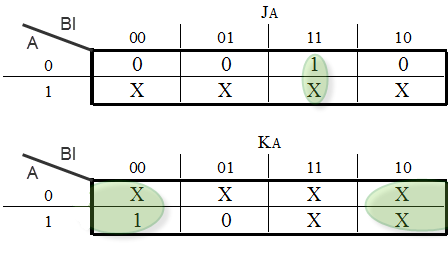Karnaugh Map for the JK - Flip Flop Input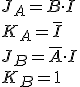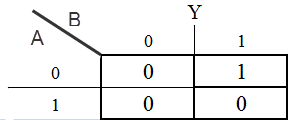Karnaugh Map for the Output variable Y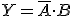Step 7

We design our circuit. We place the Flip Flops and use logic gates to form the Boolean functions that we calculated. The gates take input from the output of the Flip Flops and the Input of the circuit. Don't forget to connect the clock to the Flip Flops!

The D - Flip Flop version: (Figure below)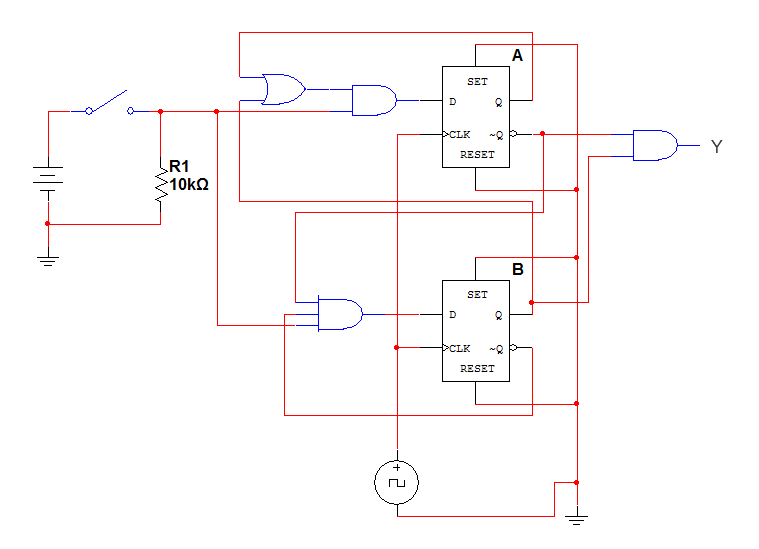The completed D - Flip Flop Sequential Circuit

The JK - Flip Flop version: (Figure below)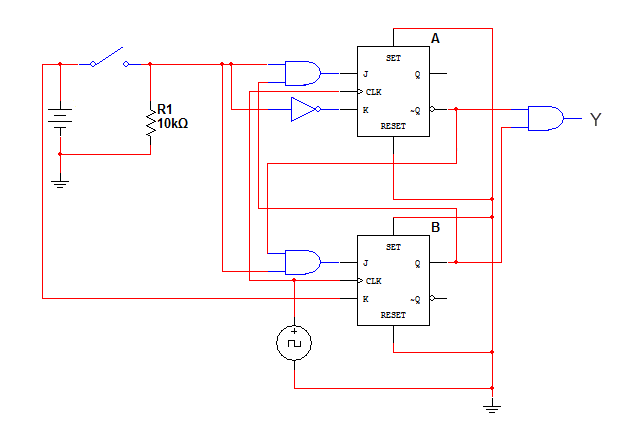The completed JK - Flip Flop Sequential Circuit

This is it! We have successfully designed and constructed a Sequential Circuit. At first it might seem a daunting task, but after practice and repetition the procedure will become trivial. Sequential Circuits can come in handy as control parts of bigger circuits and can perform any sequential logic task that we can think of. The sky is the limit! (or the circuit board, at least)

• REVIEW:
• A Sequential Logic function has a "memory" feature and takes into account past inputs in order to decide on the output.
• The Finite State Machine is an abstract mathematical model of a sequential logic function. It has finite inputs, outputs and number of states.
• FSMs are implemented in real-life circuits through the use of Flip Flops
• The implementation procedure needs a specific order of steps (algorithm), in order to be carried out.

## Contributors

Contributors to this chapter are listed in chronological order of their contributions, from most recent to first. See Appendix 2 (Contributor List) for dates and contact information.

George Zogopoulos Papaliakos (November 2010): Author of Finite State Machines section.

## Bibliography

Lessons In Electric Circuits copyright (C) 2000-2020 Tony R. Kuphaldt, under the terms and conditions of the CC BY License.